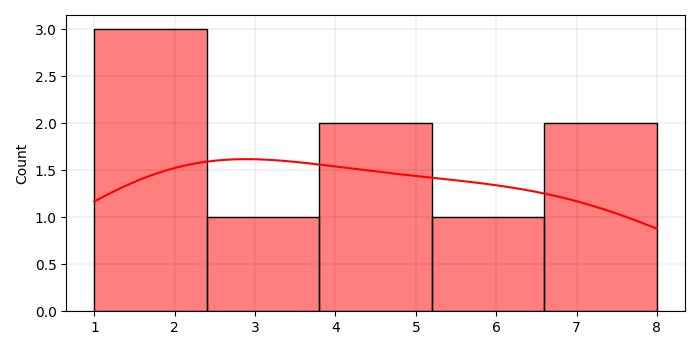# Add minor gridlines to Matplotlib plot using Seaborn

MatplotlibServer Side ProgrammingProgramming

To add minor gridlines to matplotlib plot using Seaborn, we can take the following steps −

• Create a list of numbers to plot a histogram using Seaborn.

• Plot univariate or bivariate histograms to show distributions of datasets using histplot() method.

• To make minor grid lines, we can first use major grid lines and then minor grid lines.

• To display the figure, use show() method.

## Example

import seaborn as sns
from matplotlib import pyplot as plt
plt.rcParams["figure.figsize"] = [7.00, 3.50]
plt.rcParams["figure.autolayout"] = True
x = [5, 6, 7, 2, 3, 4, 1, 8, 2]
ax = sns.histplot(x, kde=True, color='red')
ax.grid(b=True, which='major', color='black', linewidth=0.075)
ax.grid(b=True, which='minor', color='black', linewidth=0.075)
plt.show()

## Output